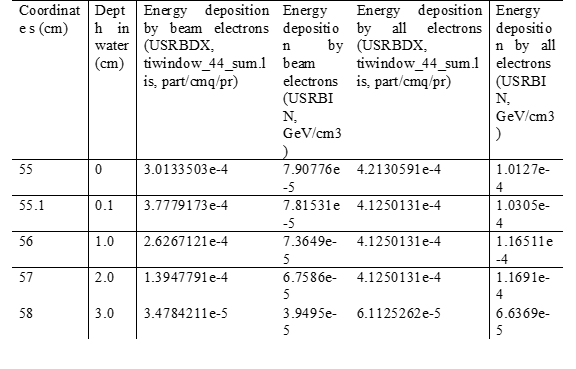# Energy comparison scored by USRBDX and USRBIN

Dear FLUKA experts,

I have one question about the deposited energy comparison scored by USRBDX and USRBIN. This question is based on my previous topic USRBDX detector area and fluence comparison to that by USRBIN.
There is an electron beam incident into the water region. Continuing with that topic, I scored the electron fluence inside the water region by different planes (USRBDX) and different bins (USRBIN). The results by USRBIN is approximately equal to the integral of the result by USRBDX. I thought it is similar for the energy deposition in the water region.
So I scored the energy deposition by USRBIN, where from my understandings I think it is the energy deposited in every gridded bin. However what is the energy scored through a plane by USRBDX? I scored that with two particle families (the beam electrons and the whole electrons). And I tabled several energy results on different locations for comparison, like the following table.The results by these two detectors are not consistent. For beam electrons, the result with USRBIN is decreasing with the water depth increasing, while the result with USRBDX is not. I guess the scored energy by USRBDX should not be the deposited energy, Do you have any idea? Thanks.
Please find the attached the input files here tiwindow.inp (41.4 KB) tiwindow.flair (56.9 KB)

Best,
Xi

Dear Xi,

from the USRBDX section of the FLUKA manual (USRBDX | FLUKA):

``````4) If the generalised particle is 208.0 (ENERGY) or 211.0 (EM-ENRGY),
the quantity scored is differential energy fluence (if
cosine-weighted) or differential energy current (energy crossing the
surface). In both cases the quantity will be expressed in GeV per
cm2 per energy unit per steradian per primary. That can sometimes
lead to confusion since GeV cm-2 GeV-1 sr-1 = cm-2 sr-1, where
energy does not appear. Note that integrating over energy and solid
angle one gets GeV/cm2.
``````

This means, that the ENERGY scorings made with USRBIN and USRBDX cannot be compared with each other, since they are different physical quantities.

Cheers,
David

Hi @horvathd ,

That makes sense, the 208.0 (ENERGY) in USRBIN is the deposited energy. Then, what is the integral of the 208.0 (ENERGY) by USRBDX (the integral can directly read by the sum.lis file)? is that the kinetic energy of the particles through the surface? Thanks.

Best,
Xi

Dear Xi,

the integral of an ENERGY current scoring by USRBDX is the kinetic energy of all particles crossing the surface (in GeV per primary). If divided by the respective area, it becomes the average kinetic energy current density through the surface [GeV/cm2].

Cheers,
David

Hi David @horvathd,

Now I got it clearly. Thank you very much!

Best,
Xi